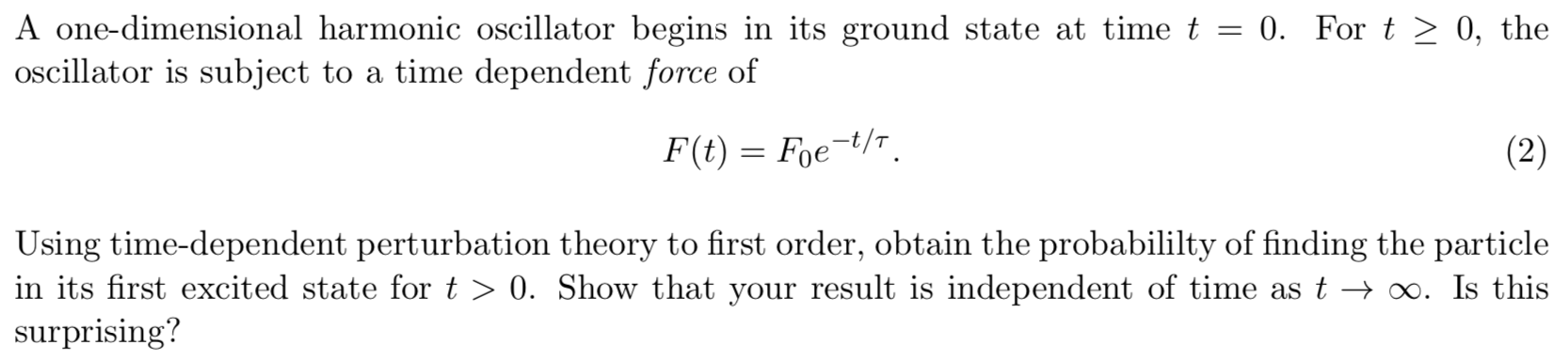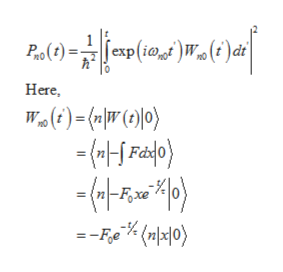# A one-dimensional harmonic oscillator begins in its ground state at time t = 0. For t > 0, theoscillator is subject to a time dependent force ofF(t) Foet/(2)Using time-dependent perturbation theory to first order, obtain the probabililty of finding the particlein its first excited state for t > 0. Show that your result is independent of time as t > o0. Is thissurprising?

Question
2 views

It's a quantum mechanics question.help_outlineImage TranscriptioncloseA one-dimensional harmonic oscillator begins in its ground state at time t = 0. For t > 0, the oscillator is subject to a time dependent force of F(t) Foet/ (2) Using time-dependent perturbation theory to first order, obtain the probabililty of finding the particle in its first excited state for t > 0. Show that your result is independent of time as t > o0. Is this surprising? fullscreen
check_circle

Step 1

Using the definition for probability from time-dependent perturbation theory,help_outlineImage Transcriptionclose)W» (i ) di Po(1) =[exp(i@ Here, Wa(t) =( \|W(})|)} -(4-*) = -Fe"* <끼지0〉 fullscreen
Step 2

In the operator notation, the position operator is,

Step 3

Using the expression for energy...

### Want to see the full answer?

See Solution

#### Want to see this answer and more?

Solutions are written by subject experts who are available 24/7. Questions are typically answered within 1 hour.*

See Solution
*Response times may vary by subject and question.
Tagged in

### Science